# Electronics and Communication Engineering - Electronic Devices and Circuits

41.
Hall effect can be used
to find type of semiconductor (whether p or n)
to find carrier concentration
to measure conductivity
all of the above
Explanation:
No answer description is available. Let's discuss.

42.
The v-i characteristic of an element is shown in below figure the element is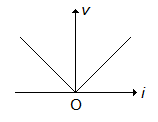non-linear, active, non-bilateral
linear, active, non-bilateral
non-linear, passive, non-bilateral
non-linear, active, bilateral
Explanation:
No answer description is available. Let's discuss.

43.
Diffusion constants Dp, Dn mobility μp, μn and absolute temperature T are related as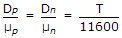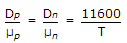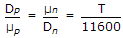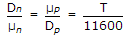Explanation:
No answer description is available. Let's discuss.

44.
The probability giving distribution of electrons over a range of allowed energy levels is known as
Maxwell's Distribution
Fermi-Dirac Distribution
Richardson Dushman Distribution
none of the above